Times up!
Times up! You may finish answering the current question and then proceed to your score!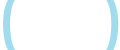Sorry, you have used all of your available hints for this lesson.
Practice Limit Reached

You've reached your daily practice limit of 12 questions.

Award InformationAwarded toQuestion HintChoose Family Member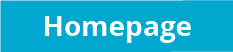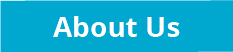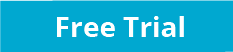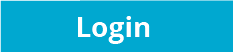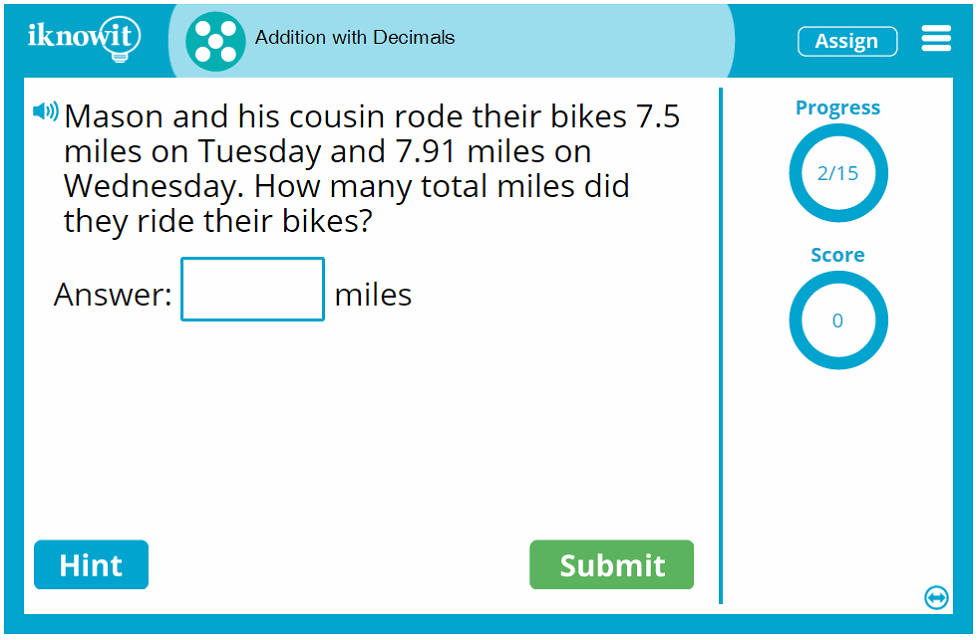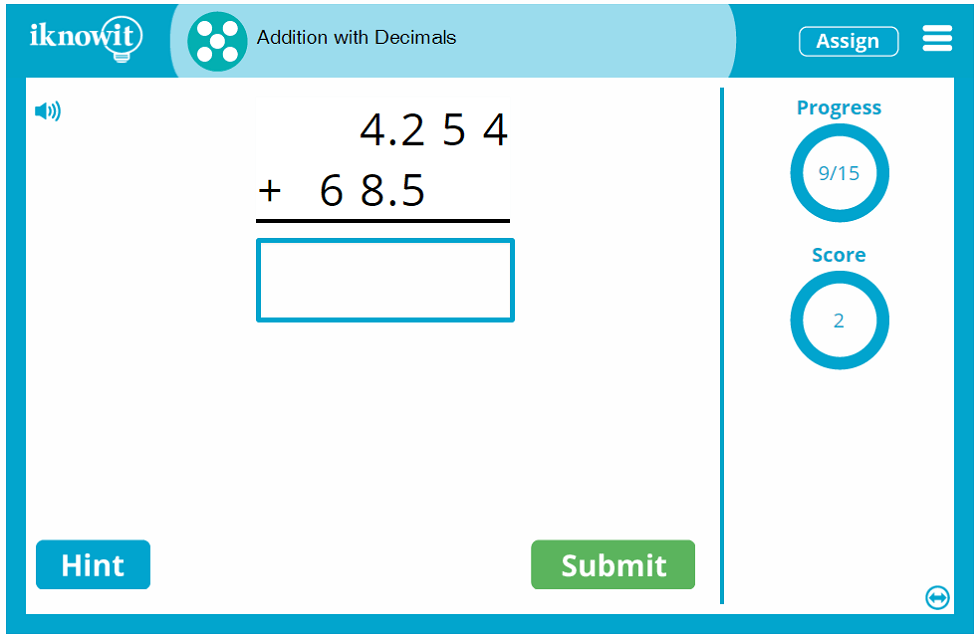## Interactive Math Practice - Addition with Decimals

In this interactive math lesson, students will practice adding multi-digit numbers with decimals. This decimals addition lesson will help students become proficient in decimal addition skills, including lining up the decimals, adding fractional parts, and adding whole numbers.

Students will be presented with a variety of question types in this adding with decimals lesson. They may be given a word problem, such as: "On average, Columbus, Ohio receives 29.12 inches of snow and Buffalo, New York receives 91.08 inches of snow annually. How many inches of snow do both cities receive annually combined?" Students will also be given horizontal addition problems and vertical addition problems. Here are some examples: "Find the sum of 46.64 and 870.084." and "98.2 + 9.05 =". As evidenced by these examples, students will be expected to align the decimal places before they begin some problems.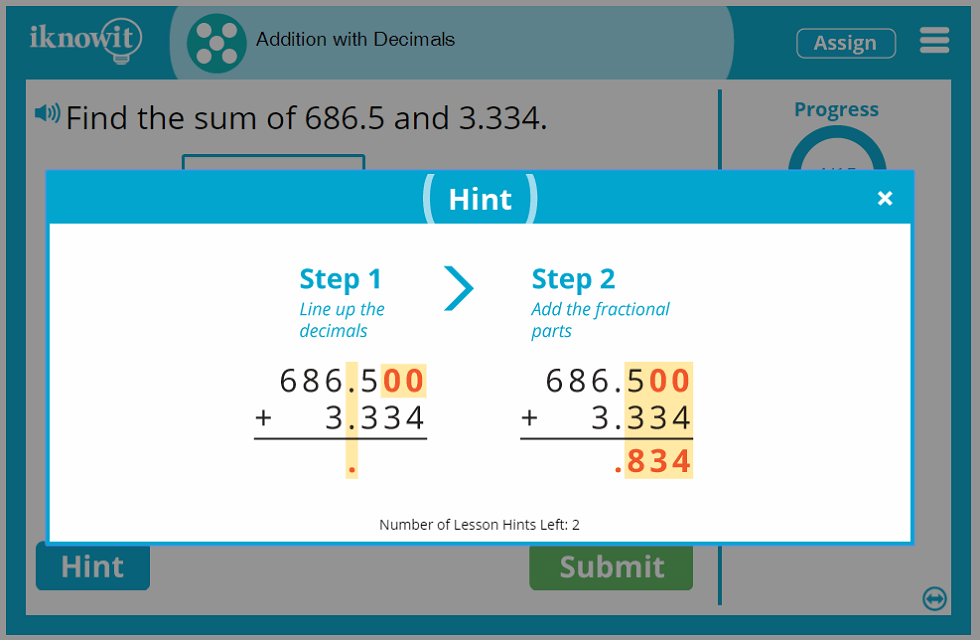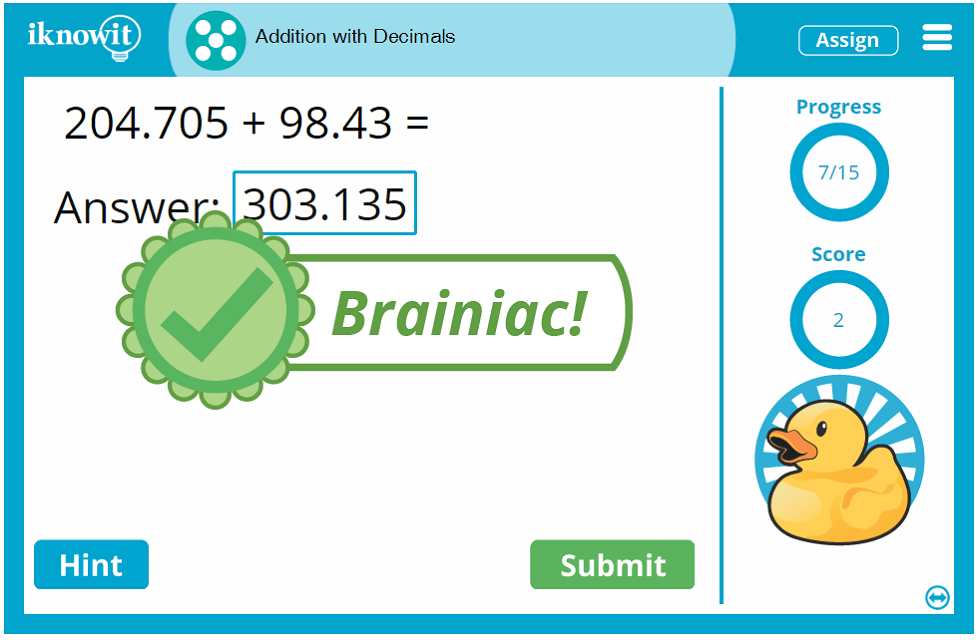iKnowIt.com is an engaging math practice website developed for elementary-aged students to help them practice basic math skills, including adding with decimals. Both teachers and students find our interactive math lessons easy and enjoyable to use. The kid-friendly layout, bright colors, whimsical graphics, and fun animations augment high quality math content covering hundreds of topics.

Students will make the most out of their math practice time in this decimal addition lesson with several unique features that set 'I Know It' math lessons apart from the rest. A score-tracker shows students how many questions they've answered correctly so far, and a progress-tracker shows them how many questions they have left to answer in the lesson. The hint button in the bottom-left corner of the screen gives students a jump-start on how to answer the question should students choose to click on it. If the students answer incorrectly, a detailed explanation page will pop up on the screen, showing the correct answer along with step-by-step instructions for how to solve the question correctly. In this addition with decimals lesson and all 'I Know It' lessons, there is always an opportunity to learn from mistakes!

In addition, you can always count on our read-aloud lesson feature, indicated by the speaker icon in the upper-left corner of the screen. When clicked, the problem will be read out loud to the student in a clear voice. This feature is ideal for students who prefer auditory learning and for ESL/ELL students.

What are you waiting for?! Go ahead and try this adding with decimals lesson with your students! We think you're going to like it! Don't forget to check out our extensive collection of other math topics!

You can sign up for a free sixty-day trial of iKnowIt.com and try out this basic addition with decimals lesson for free today! Your class may practice this math lesson for free, but they will be limited to twenty-five questions per day across all 'I Know It' math lessons. To have unlimited access, you will need to become a member of 'I Know It'.

An 'I Know It' membership comes with many benefits. Teachers are able to create a class roster and add their students, assign individual student user names and passwords, assign specific lessons to different students, view student progress and lesson scores, and change lesson settings (like limiting lesson hints and turning off the character animations).

When students log in with their unique user name and password, they will be taken to an easy-to-navigate homepage where they can get to work on the math lessons they've been given under the 'My Assignments' section. Students may also explore other lessons at their grade level, or lessons at different grade levels. Note that grade levels appear as 'Level A' or 'Level B' instead of 'Grade 1' or 'Grade 2', giving teachers the option to assign lessons based on skill level.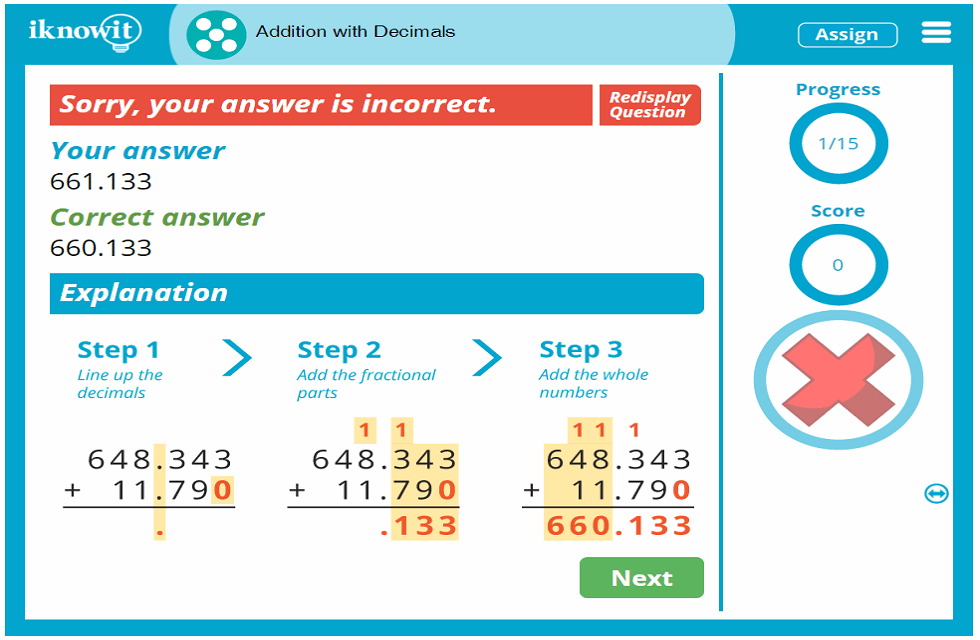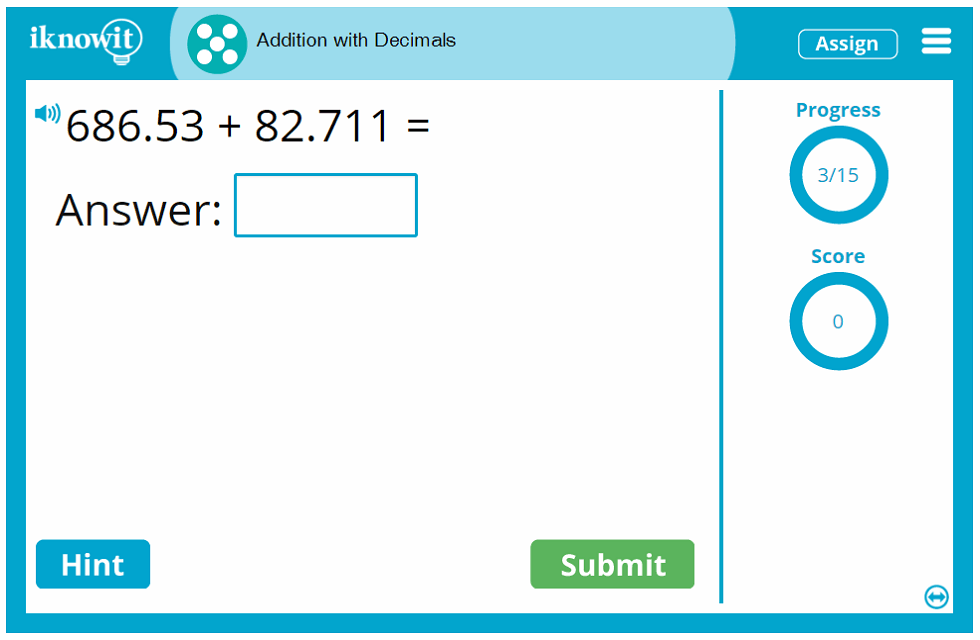## Level

This online math game is categorized on 'I Know It' as a Level E lesson. It may be ideal for fourth or fifth grade students.

## Common Core Standard

5.NBT.B.7
Numbers and Operations in Base Ten

Students will perform operations with multi-digit whole numbers and with decimals to hundredths. Specifically, students will be able to add, subtract, multiply, and divide decimals to hundredths, using drawings and strategies based on place value, order of operations, and/or the relationship between addition and subtraction. Students should relate the strategy to a written method and explain the reasonin

## You might also be interested in...

Subtraction with Decimals (Level E)
In this fifth grade-level math lesson, students will practice subtracting with decimals. They will be given subtraction word problems, vertical subtraction problems, and horizontal subtraction problems.

Addition and Subtraction with Decimals (Level E)
Students will practice adding and subtracting with decimals in this fifth grade-level math game. Students will be given addition and subtraction word problems, vertical addition and subtraction problems, and horizontal addition and subtraction problems.